# Cooperative game

A non-strategic game (see Games, theory of), defined by a triple, whereis a (usually finite) set whose elements are called players, the subsets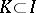are called coalitions,is a real-valued function defined on the set of coalitions, called the characteristic function of the game, andis a subset of vectors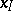(the componentscorrespond to playerin) called the imputations. Cooperative games were first introduced by J. von Neumann, 1928, as a tool in the cooperative theory of (non-cooperative) games.

In the classical theory of cooperative games one takes: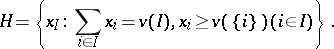On the setone introduces the binary relation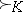of dominance (preference) of the imputations with respect to the coalition: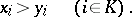Iffor some, then one writes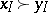. Notions of optimality of an imputation are formulated in terms of this relation of dominance.

A significant part of the contents of the theory of cooperative games consists of elaborating the notions of optimality, of proving their realizability for various special classes of cooperative games, and of actually discovering such realizations. Among the principles of optimality that have been developed in connection with cooperative games are the following: double (namely, internal and external) stability, realizable in the form of von Neumann–Morgenstern solutions (-solutions, cf. Solution in game theory); undominated imputations (see Core in the theory of games); stability with respect to threats; stability in the sense of minimization of the greatest insufficiency (see Stability in game theory); fairness (see Shapley vector); etc.

The introduction of algebraic operations in the class of cooperative games leads to the calculus of cooperative games and to the investigation of interrelations between these operations and various principles of optimality. The different special classes of cooperative games described below have been given special attention.

A simple game is a cooperative game in which the characteristic functiontakes exactly two values (usually 0 and 1); here, coalitionson which the maximum value ofis attained are called winning. A special case of simple games is a weighted majority game, in which a coalitionis winning if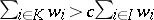, where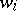(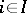) and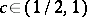are certain constants.

A balanced game is a cooperative game whose characteristic function is such that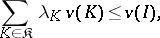if the family of coalitions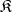and the non-negative numbers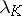(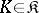) are such that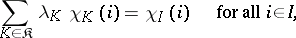where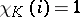ifand 0 otherwise. Balanced games and only they have a non-empty-core (cf. Core in the theory of games).

A convex game is a cooperative game whose characteristic function is such that for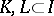,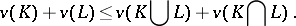(*)

In a convex game the-core is non-empty and coincides with the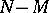-solutions. If a cooperative game is strictly convex (that is, the inequality (*) is strict), then the Shapley vector (value) is the centre of gravity of the-core.

A quota-game is a cooperative game with characteristic functionfor which there exists a vector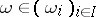such thatandfor any two players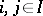().

A market game is a cooperative game induced by a market, which is taken to be a system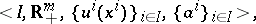whereis the set of participants in the market (withcommodities),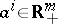is the initial bundle of commodities of the-th participant andis the utility function of the-th participant defined on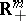. On the basis of such a market a cooperative game is constructed in whichwhile the characteristic function is defined by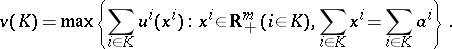The theory of classical cooperative games has undergone generalizations in various directions (see also Non-atomic game).

Games without side payments are non-strategic games defined by a triple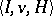, where(in contrast to classical cooperative games) is a function that associates with each coalitiona set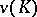of vectorssatisfying the following conditions: 1)is closed and convex; 2) if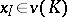and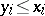(), then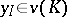; 3) if, then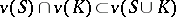; 4)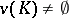for all; and 5)if and only if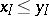for some.

Dominance in a game without side payments is defined as follows:if there exists a non-empty coalitionsuch that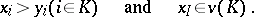A game in partition function form is a non-strategic game defined by a set of playersand a functionthat associates with each partition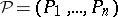of the seta vector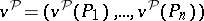. The maximal pay-off that the coalitionitself can guarantee is defined by the formula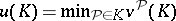. An imputation in a game in partition function form is defined as a vectorsatisfying the conditions: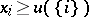();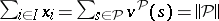for some. An imputationdominates an imputation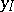with respect to a coalitionif: 1)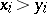(); 2); and 3) there exists a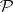such that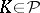and.